# The 4-colour cube

Tuesday, 30 May 2017  |  Paul Stephenson

An unmarked regular tetrahedron has planes of symmetry. It does not therefore have left- and right-handed forms. (The technical word for handedness is chirality.) But here are nets for two tetrahedra in which each face has a different colour. Focus on the blue-yellow rhombus.

In one net green lies on the left, red on the right; in the other, the order is reversed. Alternatively, focus on the central blue triangle. In one net the surrounding triangles run red-yellow-green clockwise; in the other, they do so anticlockwise. And we can analyse the completed tetrahedra in the same way.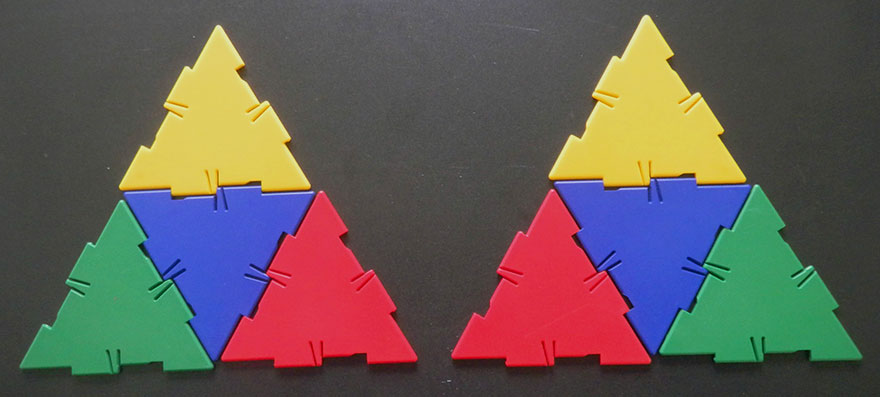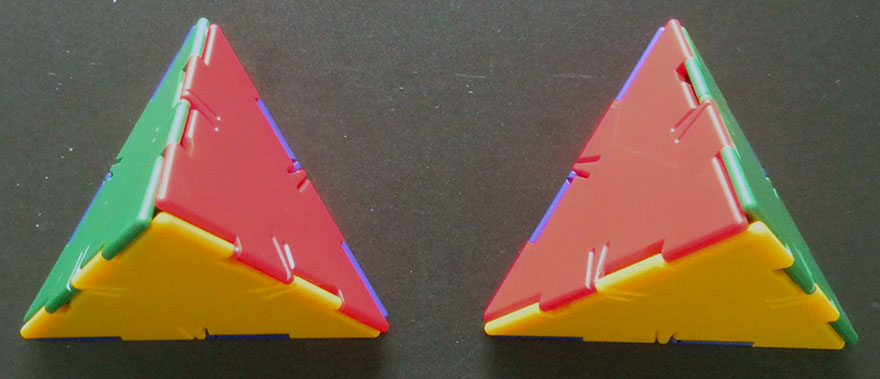The next picture shows a cube in which each corner has a different colour. One of the corners is hinged open and we see how a tetrahedron fits inside. And of course there must be two such cubes: a left-handed and a right-handed. These are the '4-colour cubes' of the title.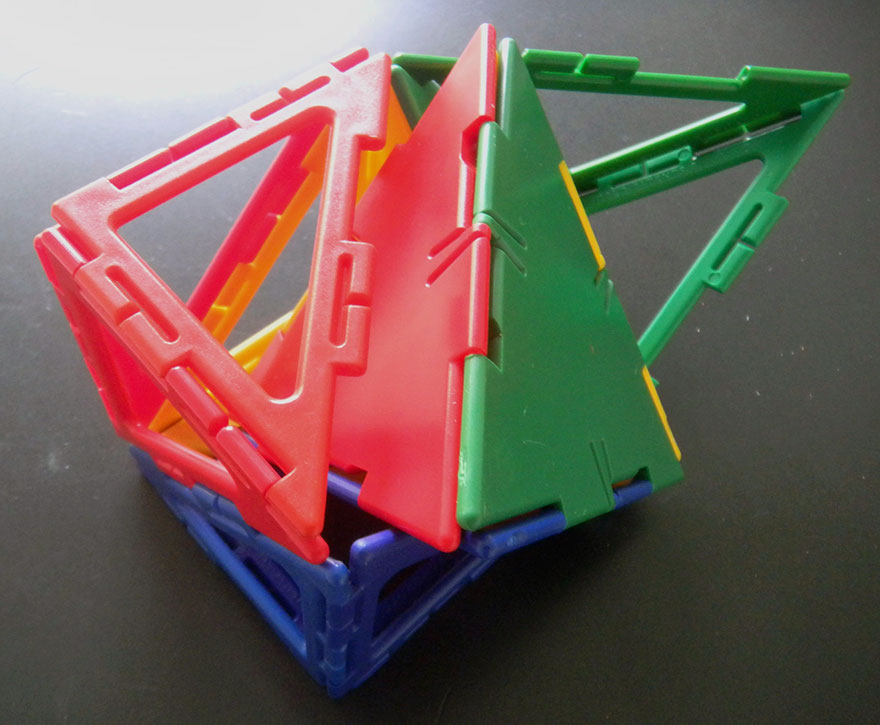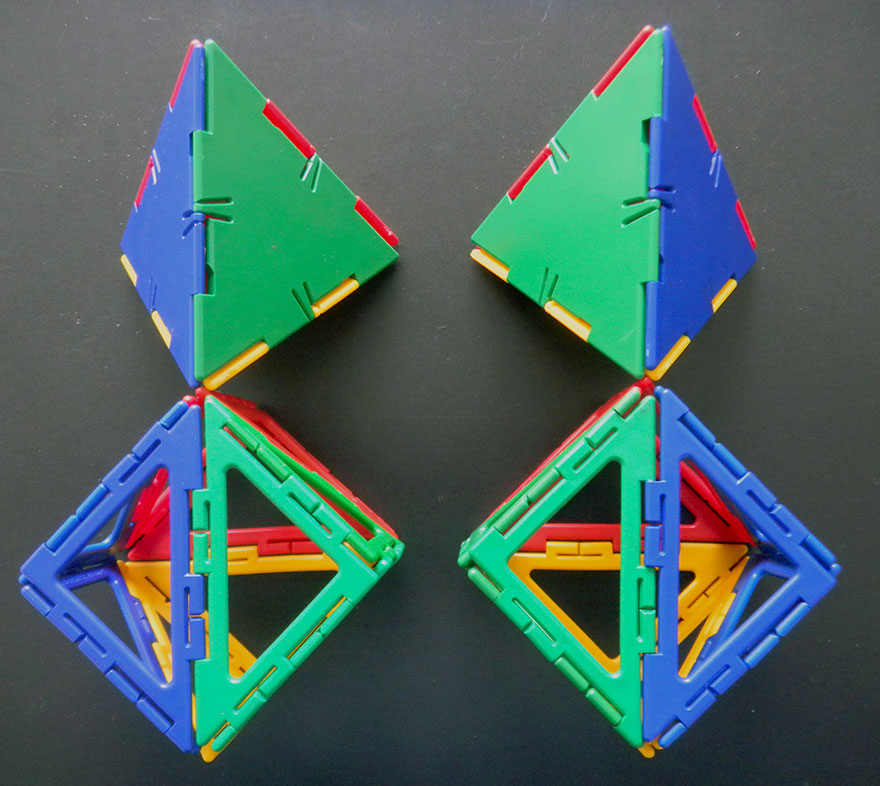Here are nets for the two 4-colour cubes: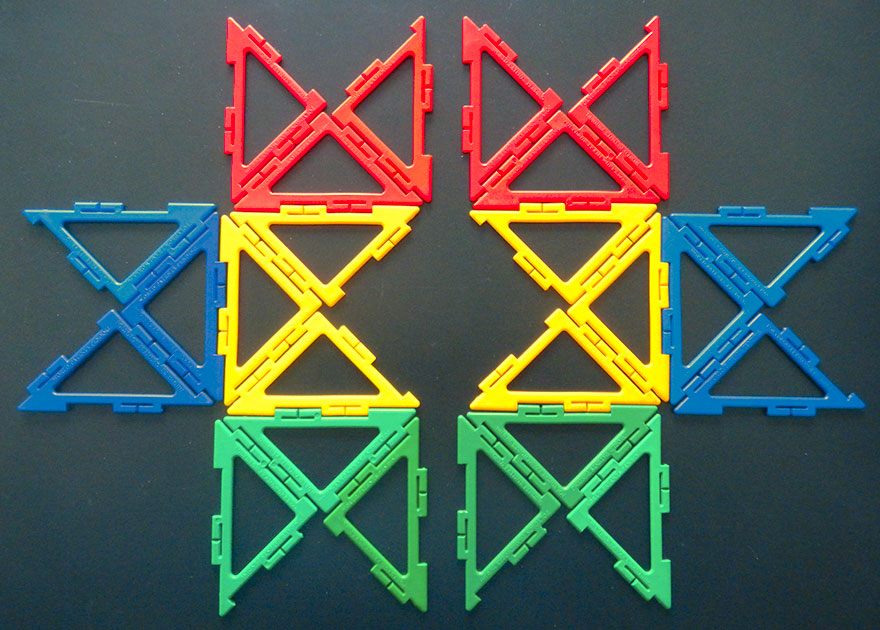In our touring maths lab, The Magic Mathworks Travelling Circus, we have a set of 64 wooden 4-colour cubes: 32 left-handed, 32 right-handed. In the picture below 1 is a 'cube-of-cubes'. There are 8. Cubes of opposite chirality share a face so there are 4 of each type. The completed cube has red corners. In 2 we separate the top and bottom layers and split each into two pairs. In 3 we flip each pair over so that what was on the bottom of each cube is now on the top. In 4 we reassemble the cube-of-cubes. It now has blue corners.Proceeding in this way, the experimenters can complete two cubes-of-cubes of each corner colour and assemble all 8 into a 'cube-of-cubes-of-cubes':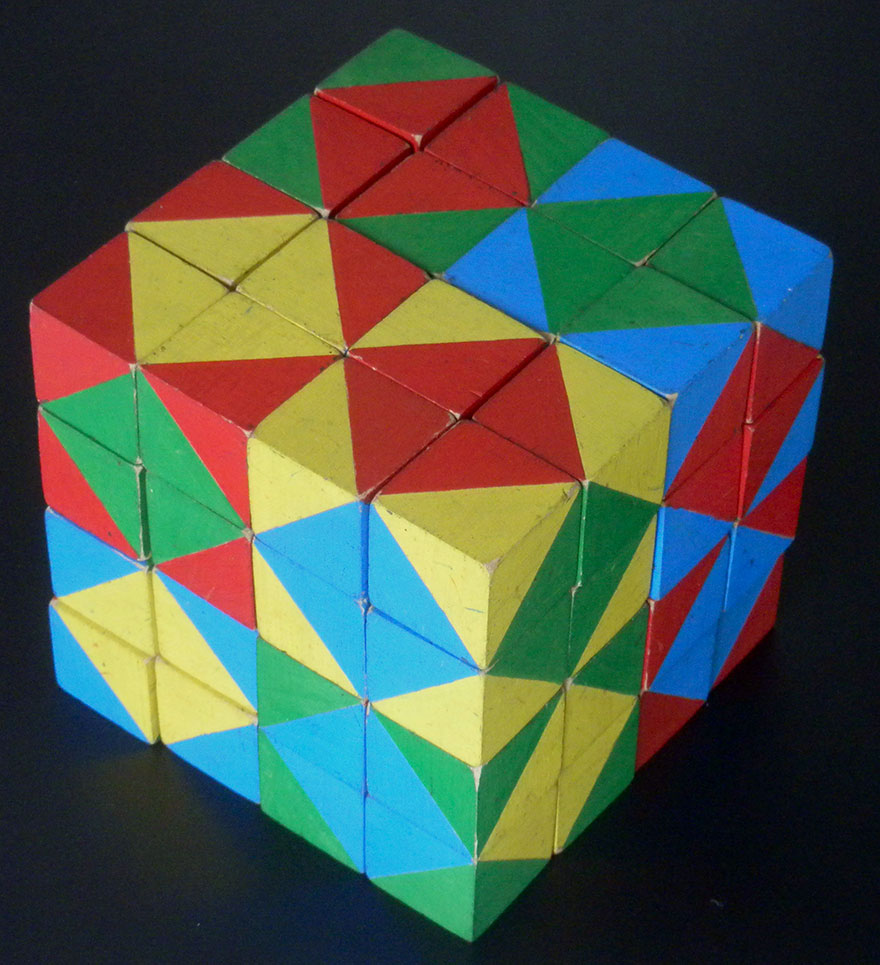From the pile of cubes all jumbled together give one person a single left-handed cube and the other a single right-handed cube. They must race to complete these two diagonally-striped walls: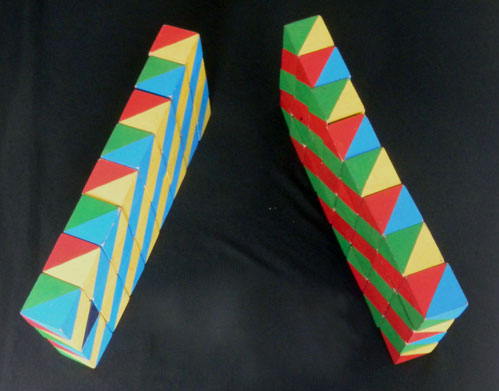The same wall seen from both sides. However, the pattern running along the top and down the edges differs in two cases. The fact is that one is made from left-handed cubes, the other from right-handed ones.

Here is a pair of 4-colour cubes in 'solid' Polydron: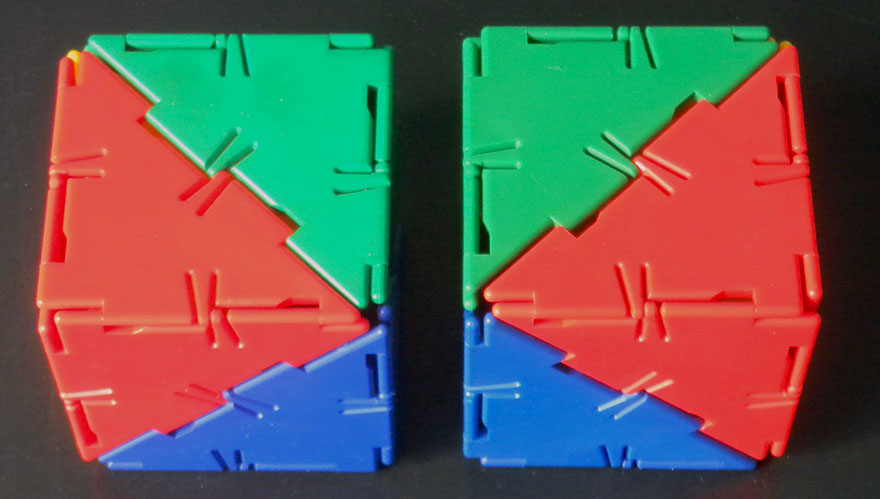If you're a teacher, I recommend you obtain enough right angle triangles (2 bulk sets) for the children to build 4 of each so that the class can do the corner-swapping exercise I described.

Paul Stephenson, Magic Mathworks Travelling Circus

 Share TweetTo contact us, call +44 (0) 1285 863980 or send us an email